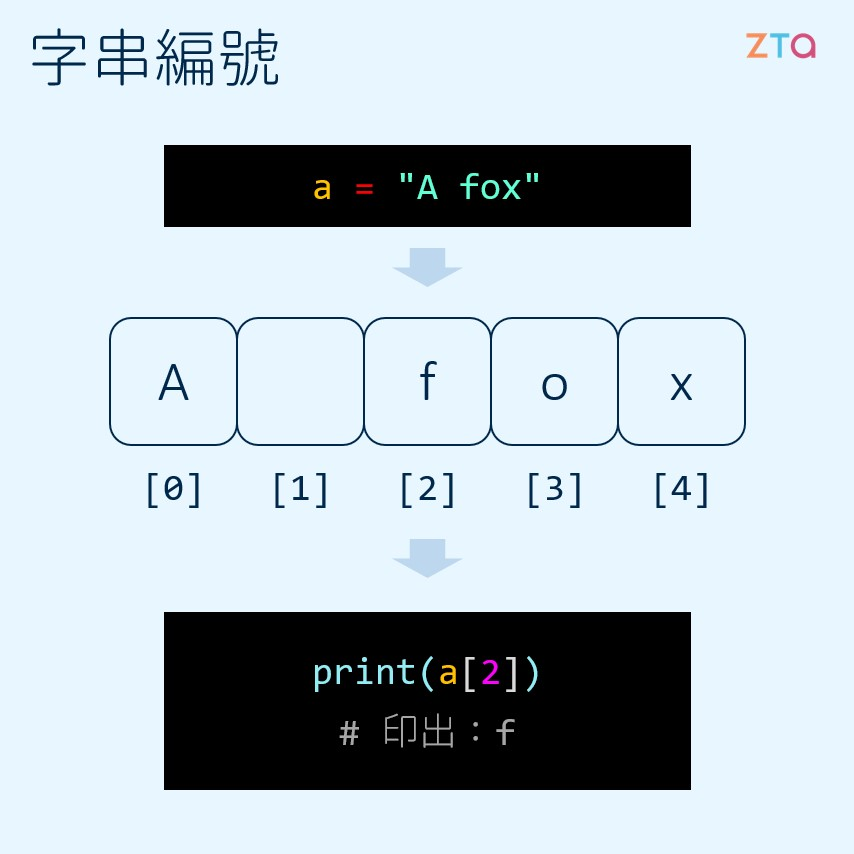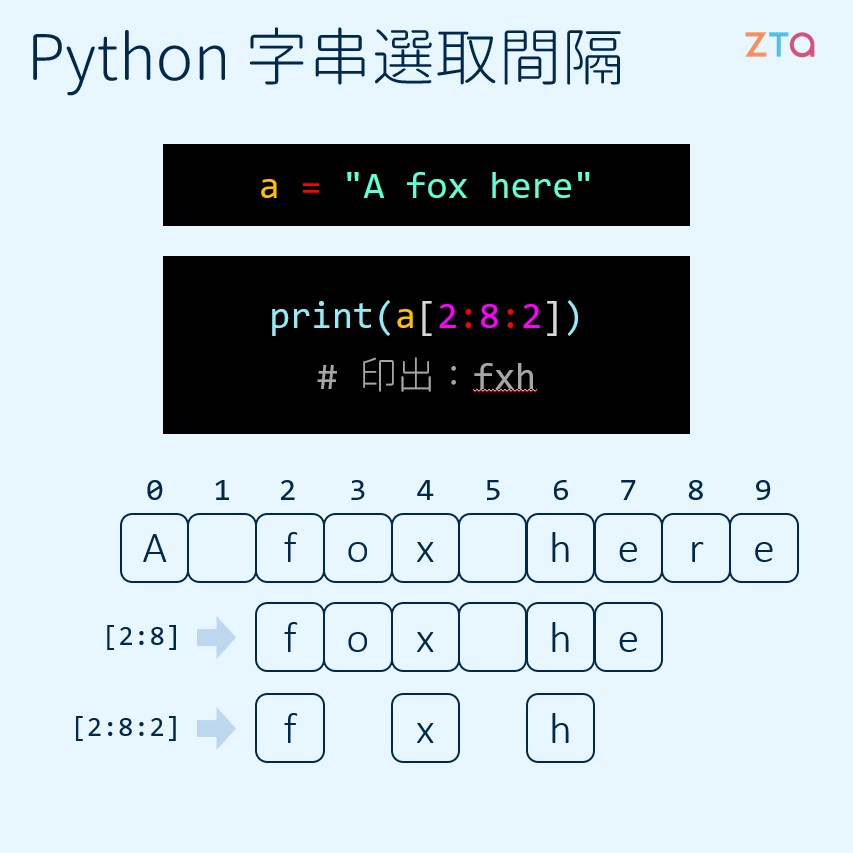字串的選取

字串的位置編號

stringA = "a fox"
print(stringA)

a fox

print(stringA)

a[x:y] 選取

[i] 可以用來獲取字串中特定位置的文字，但如果今天要獲取的是字串中的另一段字串，這個方法就不可行了。因此，Python 有另一種在字串中選取的方法，大概可以表達成 [x:y]

stringA = "a fox"

print(stringA[0:4])

a fo

1. 選取位置 x 開始至 y 結束
（包含 x，但不包含 y）

2. 選取從位置 x 開始的 (x-y) 個字元

a = "Hi there"
print(a[0:4])
print(a[:4])

Hi t
Hi t

b = "Hello there"
print(b[4:len(b)])
print(b[4:])

o there
o there

[x:y:d] 選取

a = "ThisStringIsLong"
print(a[2:11])

isStringI

a = "ThisStringIsLong"
print(a[2:11:2])

iSrnI

a = "a fox here"
print(a[2:8:2])

fxhm = "a cool string"
print(m[::-1])

gnirts looc a

+1